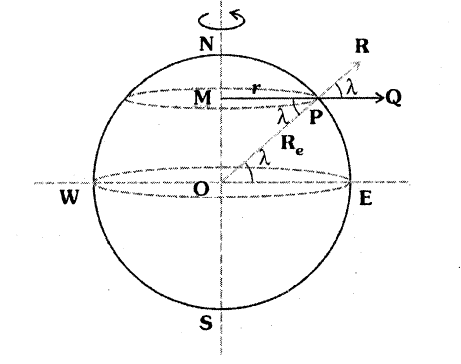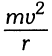# Variation in g with latitude

Hardik BatraHere the particle is performing circular motion due to the rotation of the earth. And for the circular motion it requires centripetal force then which force provides the necessary centripetal force. I think it is mgcos(lamda). Am i right?

Mentor
I think it is mgcos(lamda). Am i right?
Yes, that's correct.

•Hardik Batra
Hardik Batra
Can I do this?

mgcos(lamda) =(centrifugal force) (In PQ direction)

PR = mgcos^2(lamda)

and resultant force = mg - mgcos^2(lamda).

Cutter Ketch
No, that is incorrect. mg cos(Q) is much larger than the required centrepital force. You are forgetting about the constraint force of the ground pushing up.

For example the radius of the Earth is about 6.4e6 m. The rotational rate is once per day making the velocity about 460m/s. So V^2/R at the equator is an out 0.034 m/s^2 which is 300 X smaller than g. The normal force which would otherwise have to be = mg now is reduced to 99.7% of mg. So you weigh 0.3% less at the equator. Actually it's more than that because of the Earth's centrifugal bulge, but that's another story.

•Hardik Batra
Mentor
Can I do this

mgcos(lamda) = View attachment 109856 (centrifugal force) (In PQ direction)

PR = mgcos^2(lamda)

and resultant force = mg - mgcos^2(lamda).
No. As Cutter Ketch stated, mgcosθ is much greater than the needed centripetal force.

I was sloppy before: I just meant to say that it is the component of the weight perpendicular to the axis of rotation that provides the centripetal force, not that it equals the centripetal force.

To find the apparent g as a function of latitude (pretending a spherically symmetric earth) you'd add the centrifugal force to the force of gravity.

•Hardik Batra